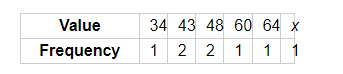# If the mode of the data: 64, 60, 48, x, 43, 48, 43, 34 is 43, then x + 3 =

Question:

If the mode of the data: 64, 60, 48, x, 43, 48, 43, 34 is 43, then x + 3 =

(a) 44
(b) 45
(c) 46
(d) 48

Solution:It is given that the mode of the given date is 43. So, it is the value with the maximum frequency.

Now, this is possible only when x = 43. In this case, the frequency of the observation 43 would be 3.

Hence,

x + 3 = 46

Hence, the correct option is (c).